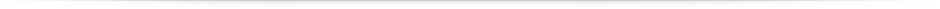# Modulating heart rate oscillation affects plasma amyloid beta and tau levels in younger and older adults

### Baseline characteristics of participants

The male and female ratio was similar across age groups and conditions (Table 1). At baseline, in neither age cohort did the Osc+ and Osc− groups differ significantly in plasma Aβ, plasma tau, age, body mass index, waist hip ratio, blood pressure, sleep, nor heart rate variability (Table 2). In addition, we compared males to females for the same baseline measures of Table 2 and reported the results in Supplementary Table. There was no significant baseline difference in CREB, bootstrap Z = 1.31, p = 0.19 (available only for 54 younger adults).

We then tested age differences in the six biomarkers (Aβ42, Aβ40, tTau, pTau-181, Aβ42/Aβ40 ratio and pTau-181/tTau ratio) at baseline. We adjusted p values to control for false discovery rate43,44. Older adults compared with younger adults showed higher baseline levels of Aβ42, t(106) = 2.51, adjusted p = 0.01, Aβ40, t(106) = 3.33, adjusted p = 0.002, pTau-181, t(76.4) = 4.95, adjusted p = 0.00004, and pTau/tTau ratios, t(71.0) = 5.65, adjusted p = 0.000006, but lower baseline Aβ42/Aβ40 ratios, t(85.0) = −3.02, adjusted p = 0.005, and no significant differences in tTau, t(106) = −0.52, adjusted p = 0.33.

### Intervention effects on Aβ42 and Aβ40

There were significant time-point by condition interactions for Aβ42, F(1, 106) = 16.62, adjusted p = 0.0005, ηp2 = 0.14, and for Aβ40, F(1, 106) = 14.01, adjusted p = 0.001, ηp2 = 0.12. Simple main effects showed that Osc+ decreased Aβ42 (p = 0.05) and Aβ40 levels (p = 0.03) from pre- to post-intervention, while Osc− increased Aβ42 (p < 0.001) and Aβ40 levels (p = 0.003). With conditions collapsed, there were no main time-point effects for either Aβ42 (p = 0.17) or Aβ40 (p = 0.50). There was no interaction for the Aβ42/Aβ40 ratio, F(1, 106) = 0.55, adjusted p = 0.26, ηp2 = 0.01, with no main time-point effect (p = 0.89). The significant results were maintained after removing outliers (Supplementary Information).

In follow-up tests where we split the data (N = 108) into the younger (N = 54) and older (N = 54) age groups (Fig. 1), the time-point by condition interactions on Aβ levels remained significant for younger adults, F(1, 52) = 12.30, adjusted p = 0.002, ηp2 = 0.19 for Aβ42 and F(1, 52) = 6.64, adjusted p = 0.01, ηp2 = 0.11 for Aβ40 as well as for older adults, F(1, 52) = 4.51, adjusted p = 0.04, ηp2 = 0.08 for Aβ42 and F(1, 52) = 7.37, adjusted p = 0.01, ηp2 = 0.12 for Aβ40. For the Aβ42/Aβ40 ratio, older adults showed a trend towards an interaction, F(1, 52) = 3.35, adjusted p = 0.05, ηp2 = 0.06, whereas younger adults showed no interaction effect, F(1, 52) = 0.02, adjusted p = 0.45, ηp2 < 0.001.

### Intervention effects on tTau and pTau-181

Across all participants, there were no significant time-point by condition interactions for either tTau, F(1, 106) = 2.01, adjusted p = 0.10, ηp2 = 0.02 or pTau-181, F(1, 106) = 3.28, adjusted p = 0.05, ηp2 = 0.03. However, the pTau/tTau ratio showed a significant interaction, F(1, 106) = 7.41, adjusted p = 0.01, ηp2 = 0.07. Simple main effects showed that Osc− decreased the pTau/tTau ratio (p = 0.02) from pre- to post-intervention, while Osc+ increased it but not significantly (p = 0.14). With conditions collapsed, there were no main time-point effects (p = 0.49).

Based on our hypothesis that changes in the Aβ42/Aβ40 ratio and the pTau/Tau ratio could both be affected by changes in brain clearance, we expected some correlation in change among these two ratio scores. Changes in the pTau/Tau ratio correlated in the positive direction with changes in the Aβ42/Aβ40 ratio when controlled for their baseline values across all participants, r(104) = 0.20, adjusted p = 0.04. However, the correlation between ratio scores was not significant independently for younger adults, r(50) = 0.20, adjusted p = 0.10, nor for older adults, r(50) = 0.20, adjusted p = 0.10.

### Relationship between changes in plasma Aβ levels and noradrenergic-related gene transcription activity

Among the younger subset tested (N = 54), there was a significant time-point by condition interaction in CREB transcription factor activity, bootstrap Z = −3.30, adjusted p = 0.002. While Osc+ showed no significant changes (Z = −0.45, p = 0.65), Osc− increased CREB activity after intervention (Z = 2.70, p = 0.008). When we examined the correlations between changes in CREB activity and changes in the six biomarkers, we found that individual decreases in plasma Aβ40 from pre- to post-intervention were associated with decreases in activity of the CREB transcription factor that mediates sympathetic nervous system-induced β-adrenergic signaling (0.75-fold prevalence of CREB response elements in Aβ40 down-regulated vs. up-regulated genes; 0.418 log2 ratio units ± 0.120 standard error; Z = 2.98, adjusted p = 0.002). Decreases in CREB activity were not significantly associated with individual decreases in plasma Aβ42 from pre- to post-intervention (0.87-fold; 0.207 ± 0.114; Z = 1.82, adjusted p = 0.05) and thus showed an inverse association with the Aβ42/Aβ40 ratio (1.32-fold; −0.392 ± 0.116; Z = −3.39, adjusted p = 0.002). Increases in pTau-181 were associated with decreased CREB activity (1.35-fold; −0.427 ± 0.120; Z = −3.56, adjusted p = 0.002). Neither tTau (1.18-fold; −0.237 ± 0.136; Z = −1.75, adjusted p = 0.06), nor pTau-181/tTau ratios (1.06-fold; −0.083 ± 0.101; Z = −0.81, adjusted p = 0.24) showed a significant association with CREB activity.

### Individual differences in plasma Aβ42/40 and negative affect

There was no intervention effect for negative affect, F(1, 50) = 0.32, p = 0.58, ηp2 = 0.01 for younger adults and F(1, 45) = 0.73, p = 0.40, ηp2 = 0.02 for older adults. However, pre- to post-intervention increases in Aβ42/40 ratio were associated with decreases in negative affect, r(43) = −0.41, adjusted p = 0.007 for older adults, but not for younger adults, r(48) = −0.02, adjusted p = 0.45.

### Intervention effects on other physiological variables

Other than plasma Aβ and tau changes, we did not see significant intervention-induced changes in any other physiological measures including BMI, blood pressure, RMSSD (root mean square of successive differences) at rest, total sleep time, REM (rapid eye movement) sleep, deep sleep, and RMSSD during deep sleep (all p’s > 0.05, Table 3).